Search for on the web shopping
Mon, 26 Sep, 2022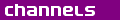Top links - Sudoku - Collectibles - PSP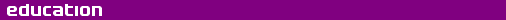In physics, the photon (from Greek φως, "phōs", meaning light) is the quantum of the electromagnetic field, for instance light. The term photon was coined by Gilbert Lewis in 1926.The photon can be perceived as a wave or a particle, depending on how it is measured The photon is one of the elementary particles. Its interactions with electrons and atomic nuclei account for a great many of the features of matter, such as the existence and stability of atoms, molecules, and solids. These interactions are studied in quantum electrodynamics (QED), which is the oldest part of the Standard Model of particle physics.In some respects a photon acts as a particle, for instance when registered by the light sensitive device in a camera. In other respects, a photon acts like a wave, as when passing through the optics in a camera. According to the so-called wave-particle duality in quantum physics, it is natural for the photon to display either aspect of its nature, according to the circumstances. Normally, light is formed from a large number of photons, with the intensity related to the number of them. At low intensity, it requires very sensitive instruments, used in astronomy or spectroscopy, for instance, to detect the individual photons.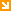Jump to Page Contents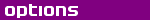Pay as you go No monthly charges. Access for the price of a phone call Go> Unmetered Flat rate dialup access from only £4.99 a month Go> Broadband Surf faster from just £13.99 a month Go>

 Save Even More Combine your phone and internet, and save on your phone calls More Info>

 This weeks hot offer24: Series 5 In association with Amazon.co.uk £26.97

ContentsSymbolPropertiesPhotons in vacuoPhotons in matterSymbol - Contents

A photon is usually given the symbol$, gamma$, the Greek letter gamma, although in nuclear physics this symbol refers to a very high-energy photon (a gamma ray).Properties - Contents

Photons are commonly associated with visible light, but this is actually only a very limited part of the electromagnetic spectrum. All electromagnetic radiation is quantized as photons: that is, the smallest amount of electromagnetic radiation that can exist is one photon, whatever its wavelength, frequency, energy, or momentum, and that light or fields interact with matter in discrete units of one or several photons. Photons are fundamental particles. They can be created and destroyed when interacting with other particles, but are not known to decay on their own.A photon of a definite frequency is not a localized particle. Photons thus exhibit a position/frequency uncertainty relation similar to that of matter particles and exactly analogous to the bandwidth theorem of classical optics. Photons have zero mass and zero electric charge, but they do carry energy, momentum and angular momentum. Photons are always moving, and photons in a vacuum always move at a constant speed with respect to all observers, even when those observers are themselves moving. This speed is called the vacuum speed of light. The energy and momentum carried by a photon is proportional to its frequency (or inversely proportional to its wavelength). This momentum can be transferred when a photon interacts with matter. The force due to a large number of photons falling on a surface is known as radiation pressure, which may be used for propulsion with a solar sail.The actual upper limit of the photon rest mass compatible with observations depends on additional assumptions. A conservative consensus limit of the Particle Data Group is 6×10-17 eV based on changes in magnetohydrodynamics which would contradict solar wind observations.Photons are deflected by a gravitational field twice as much as Newtonian mechanics predicts for a mass traveling at the speed of light with the same momentum as the photon. This observation is commonly cited as evidence supporting Einstein's theory of gravitation, general relativity. In general relativity, photons always travel in a "straight" line, after taking into account the curvature of spacetime. (In curved space, such lines are called geodesics).

Creation
Photons are produced by atoms when a bound electron moves from one orbital to another orbital with less energy. Photons can also be emitted by an unstable nucleus when it undergoes some types of nuclear decay. Furthermore, photons are produced whenever charged particles are accelerated.Atoms continuously emit photons due to their collisions with each other. The wavelength distribution of these photons is thus related to their absolute temperature. The Planck distribution determines the probability of a photon being a certain wavelength when emitted by a collection of atoms at a given temperature. The spectrum of such photons is normally peaked in the range between microwave and infrared, but sufficiently hot objects (such as the surface of the Sun or a lightbulb filament) will emit visible light as well. As temperature is further increased, some photons will reach even higher frequencies, such as ultraviolet and X-ray.Radio, television, radar and other types of transmitters used for telecommunication and remote sensing routinely create a wide variety of low-energy photons by the oscillation of electric fields in conductors. Magnetrons emit coherent photons used in household microwave ovens. Klystron tubes are used when microwave emissions must be more finely controlled. Masers and lasers create monochromatic photons by stimulated emission. More energetic photons can be created by nuclear transitions, particle-antiparticle annihilation, and in high-energy particle collisions.

Spin
Photons have spin 1, and they are therefore classified as bosons. Photons mediate the electromagnetic interaction; they are the gauge bosons of quantum electrodynamics (QED), which is a U(1) gauge theory. A non- relativistic spin-1 particle has three possible spin states (−1, 0 and +1). However, in the framework of special relativity, this is not the case for massless spin-1 particles, such as the photons, which have only two spin projections, helicities, corresponding to the right- and left-handed circular polarizations of classical electromagnetic waves. The more familiar linear polarization is formed by a superposition of the two spin projections of a photon.

Quantum state
Visible light from ordinary sources (like the Sun or a lamp) is a mixture of many photons of different wavelengths. One sees this in the frequency spectrum, for instance by passing the light through a prism. In so-called "mixed states", which these sources tend to produce, light can consist of photons in thermal equilibrium (so-called black-body radiation). Here they in many ways resemble a gas of particles. For example, they exert pressure, known as radiation pressure.On the other hand, an assembly of photons can also exist in much more well-organized coherent states, such as in the light emitted by an ideal laser. The high degree of precision obtained with laser instruments is due to this organization.The quantum state of a photon assembly, like that of other quantum particles, is the so-called Fock state denoted$|nrangle$, meaning$, n$ photons in one of the distinct "modes" of the electromagnetic field. If the field is multimode (involves several different wavelength photons), its quantum state is a tensor product of photon states, for example:$|n_{k_0}rangleotimes|n_{k_1}rangleotimesdotsotimes|n_{k_n}rangledots$
Here$, k_i$ denote the possible modes, and$, n_{k_i}$ the number of photons in each mode

Molecular absorption
A typical molecule,$, M$, has many different energy levels. When a molecule absorbs a photon, its energy is increased by an amount equal to the energy of the photon. The molecule then enters an excited state,$, M^*$.$M + gamma to M^* ,$Photons in vacuo - Contents

In empty space ( vacuum) all photons move at the speed of light, c, defined as 299,792,458 metres per second, or approximately 3×108 m/s. The metre is defined as the distance travelled by light in a vacuum in 1/299,792,458 of a second, so the speed of light does not suffer any experimental uncertainty, unlike the metre or the second, which rely on the second being defined by means of a very accurate clock.According to one principle of Einstein's special relativity, all observations of the speed of light in vacuo are same in all directions to any observer in an inertial frame of reference. This principle is generally accepted in physics since many practical consequences for high-energy particles in theoretical and experimental physics have been observed.Photons in matter - Contents

When photons pass through matter, such as a prism, different frequencies will be transmitted at different speeds. This is called dispersion of colors, where photons of different frequencies exit at different angles. A similar phenomenon occurs in reflection where surfaces can reflect photons of various frequencies at different angles.The associated dispersion relation for photons is a relation between frequency,$, f$ , and wavelength,$, lambda$, or equivalently, between their energy,$, E$, and momentum,$, p$. It is simple in vacuo, since the speed of the wave,$, v$, is given by$v=lambda f = c,$
The photon quantum relations are:$E=hf ,$ and$p=h/lambda ,$
Here$, h$ is Planck's constant. So one can also write the dispersion relation as$E=pc ,$
which is characteristic of a zero-mass particle. One sees how remarkably Planck's constant relates the wave and particle aspects.In a material, photons couple to the excitations of the medium and behave differently. These excitations can often be described as quasi-particles (such as phonons and excitons); that is, as quantized wave- or particle-like entities propagating though the matter. "Coupling" means here that photons can transform into these excitations (that is, the photon gets absorbed and medium excited, involving the creation of a quasi-particle) and vice versa (the quasi-particle transforms back into a photon, or the medium relaxes by re-emitting the energy as a photon). However, as these transformations are only possibilities, they are not bound to happen and what actually propagates through the medium is a polariton; that is, a quantum-mechanical superposition of the energy quantum being a photon and of it being one of the quasi-particle matter excitations.According to the rules of quantum mechanics, a measurement (here: just observing what happens to the polariton) breaks this superposition; that is, the quantum either gets absorbed in the medium and stays there (likely to happen in opaque media) or it re-emerges as photon from the surface into space (likely to happen in transparent media).Matter excitations have a non-linear dispersion relation; that is, their momentum is not proportional to their energy. Hence, these particles propagate slower than the vacuum speed of light. (The propagation speed is the derivative of the dispersion relation with respect to momentum.) This is the formal reason why light is slower in media (such as glass) than in vacuum. (The reason for diffraction can be deduced from this by Huygens' principle.) Another way of phrasing it is to say that the photon, by being blended with the matter excitation to form a polariton, acquires an effective mass, which means that it cannot travel at c, the speed of light in a vacuum.
Change Text Size:
[A] [default] [A]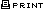| |